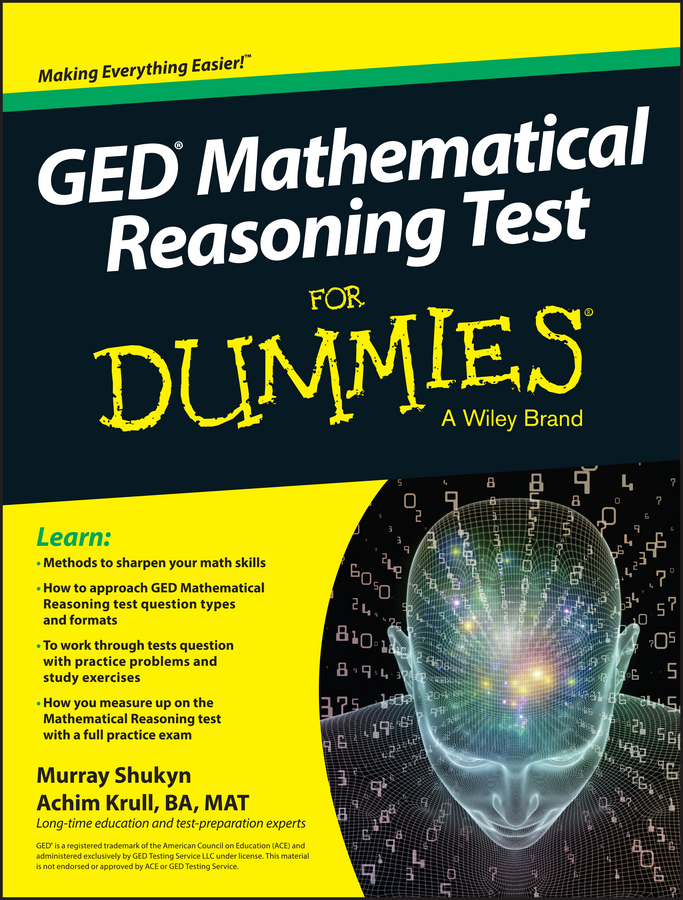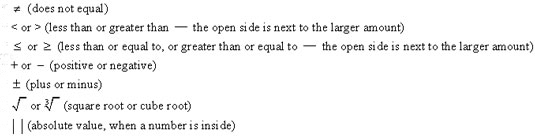##### GED Mathematical Reasoning Test For DummiesYour math tool kit for the PSAT/NMSQT — techniques that allow you to cut through problems like a hot knife through butter — contains some tricks that are especially useful for algebra and function questions. Read on:

• Be sure you know what the symbols mean. Algebra often deals with equations (the equal sign is a dead giveaway), but you may also see these little signs:• Don’t forget your calculator when you’re solving algebra and function problems. These questions often force you to deal with exponents and square roots, which most calculators can handle easily.

• Try plugging in. Pick a likely number, but not just any number, and plug it in where you see a variable. Doing so should give you an idea of the answer or allow you to check an answer you’ve already figured out.

• Use backsolving. Take one of the answers — Choice (C) is often a good place to start — and substitute that answer in the equation. Then check to see which answer works.

• In equations, isolate what you need to solve. After you know what you’re supposed to find (the value of x perhaps), isolate that variable on one side of the equation. By definition, each side of an equation is equal, so if the variable is all alone on one side, the other side represents its value.